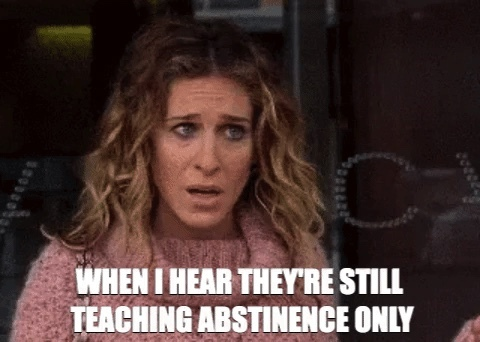Like   Tweet   Pin   +1   in
 table div table+table div table{width:100%;padding:0}table div table+table div table img{width:96.23%;padding:0;float:none}table div table+table div table td{width:100%;padding:0 1.88% 18px}/* styles *//* styles */ "Education is the ability to meet life’s situations." - Dr. John G. Hibben "Knowledge is power. Information is liberating. Education is the premise of progress, in every society, in every family." – Kofi Annan
 table div table+table+table+table div table{width:100%;padding:0}table div table+table+table+table div table img{width:96.23%;padding:0;float:none}table div table+table+table+table div table td{width:100%;padding:0 1.88% 18px}/* styles */table div table+table+table+table+table+table div table{width:100%;padding:0}table div table+table+table+table+table+table div table img{width:96.23%;padding:0;float:none}table div table+table+table+table+table+table div table td{width:100%;padding:0 1.88% 18px}/* styles */# Know

May is#SexEdForAll month. This SIECUS initiative stems from the former Teen Pregnancy Prevention Month. You see, there is only one way to prevent pregnancy, and that is sex education. If you are not educated about prevention, you don't have the tools to practice safer sex. Safer sex includes everything from abstinence to effective and affordable birth control. These skills are taught within the comprehensive sex education framework. In fact, it takes a team to ensure the information given to young people is uniform across the states.

I recently published a piece on Medium about this very topic. Hey, Can We Talk? Why parents must embrace their role as sex educator.

 table div table+table+table+table+table+table+table+table div table{width:100%;padding:0}table div table+table+table+table+table+table+table+table div table img{width:96.23%;padding:0;float:none}table div table+table+table+table+table+table+table+table div table td{width:100%;padding:0 1.88% 18px}/* styles */When Your Son Asks You About the Furries, It's Time for the Sex Talk by Chris L. Robinson.

 table div table+table+table+table+table+table+table+table+table+table div table{width:100%;padding:0}table div table+table+table+table+table+table+table+table+table+table div table img{width:96.23%;padding:0;float:none}table div table+table+table+table+table+table+table+table+table+table div table td{width:100%;padding:0 1.88% 18px}/* styles */table div table+table+table+table+table+table+table+table+table+table+table div table{width:100%;padding:0}table div table+table+table+table+table+table+table+table+table+table+table div table img{width:96.23%;padding:0;float:none}table div table+table+table+table+table+table+table+table+table+table+table div table td{width:100%;padding:0 1.88% 18px}/* styles */# Watch

Watch as I talk about #SexEdForAll

I just can't get enough of this family. In Illinois it is officially summer, so this song certainly made me smile! Enjoy this mental break with your kids.

# Support

Many homeless shelters and domestic abuse shelters are in need of feminine hygiene products and diapers. Consider purchasing a bunch and dropping off the supplies -- after calling first, of course! If you'd rather donate money, Period.org is a great place to start.

If you live in the far west Chicago 'burbs, another great group isGoWithTheFlow.org.

 table div table+table+table+table+table+table+table+table+table+table+table+table+table+table div table{width:100%;padding:0}table div table+table+table+table+table+table+table+table+table+table+table+table+table+table div table img{width:96.23%;padding:0;float:none}table div table+table+table+table+table+table+table+table+table+table+table+table+table+table div table td{width:100%;padding:0 1.88% 18px}/* styles */# Subscribe

Do you have children in third to sixth grade? There is a great newsletter written by two delightful, witty girls geared for that audience. The newsletters are uplifting and fun, and also offer suggestions on how to navigate the world right now. Check it out here: The Founding Kids.

It has nothing to do with sex and everything to do with keeping a positive attitude.

 table div table+table+table+table+table+table+table+table+table+table+table+table+table+table+table+table div table{width:100%;padding:0}table div table+table+table+table+table+table+table+table+table+table+table+table+table+table+table+table div table img{width:96.23%;padding:0;float:none}table div table+table+table+table+table+table+table+table+table+table+table+table+table+table+table+table div table td{width:100%;padding:0 1.88% 18px}/* styles */# Resources

Centers for Disease Control and Prevention
 table div table+table+table+table+table+table+table+table+table+table+table+table+table+table+table+table+table+table div table{width:100%;padding:0}table div table+table+table+table+table+table+table+table+table+table+table+table+table+table+table+table+table+table div table img{width:96.23%;padding:0;float:none}table div table+table+table+table+table+table+table+table+table+table+table+table+table+table+table+table+table+table div table td{width:100%;padding:0 1.88% 18px}/* styles */table div table+table+table+table+table+table+table+table+table+table+table+table+table+table+table+table+table+table+table+table+table div table{width:100%;padding:0}table div table+table+table+table+table+table+table+table+table+table+table+table+table+table+table+table+table+table+table+table+table div table img{width:96.23%;padding:0;float:none}table div table+table+table+table+table+table+table+table+table+table+table+table+table+table+table+table+table+table+table+table+table div table td{width:100%;padding:0 1.88% 18px}/* styles */table div table+table+table+table+table+table+table+table+table+table+table+table+table+table+table+table+table+table+table+table+table+table+table div table{width:100%;padding:0}table div table+table+table+table+table+table+table+table+table+table+table+table+table+table+table+table+table+table+table+table+table+table+table div table img{width:96.23%;padding:0;float:none}table div table+table+table+table+table+table+table+table+table+table+table+table+table+table+table+table+table+table+table+table+table+table+table div table td{width:100%;padding:0 1.88% 18px}/* styles */